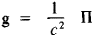# Poynting Vector

(redirected from Poynting flux)

## Poynting vector

[′pȯint·iŋ ‚vek·tər]
(electromagnetism)
A vector, equal to the cross product of the electric-field strength and the magnetic-field strength (mks units) whose outward normal component, when integrated over a closed surface, gives the outward flow of electromagnetic energy through that surface.
McGraw-Hill Dictionary of Scientific & Technical Terms, 6E, Copyright © 2003 by The McGraw-Hill Companies, Inc.
The following article is from The Great Soviet Encyclopedia (1979). It might be outdated or ideologically biased.

## Poynting Vector

the vector of the flux density of electromagnetic energy; named after the English physicist J. H. Poynting (1852–1914).

The magnitude of the Poynting vector is equal to the energy transferred per unit time through a unit of surface perpendicular to the direction of propagation of the electromagnetic energy, that is, to the direction of the Poynting vector. In the absolute (Gaussian) system of units, Π = (e/π)[EH], where [EH] is the vector product of the intensities of the electric flux E and magnetic field H and c is the speed of light in a vacuum; in the International System, Π = [EH]. The flow of the Poynting vector through a closed surface bounding a system of charged particles gives the value of the energy lost by the system per unit time as a result of the emission of electromagnetic waves. The momentum density of an electromagnetic field g is expressed in terms of the Poynting vector:G. IA. MIAKISHEV

The Great Soviet Encyclopedia, 3rd Edition (1970-1979). © 2010 The Gale Group, Inc. All rights reserved.
Site: Follow: Share:
Open / Close